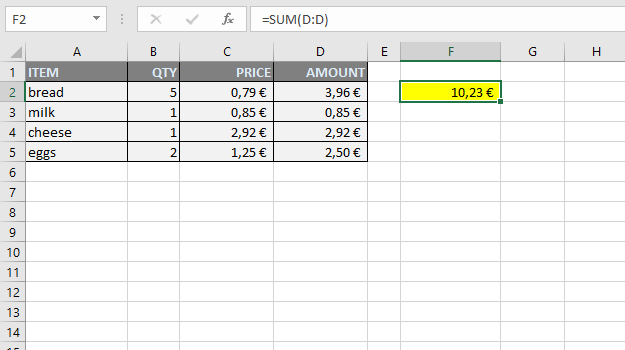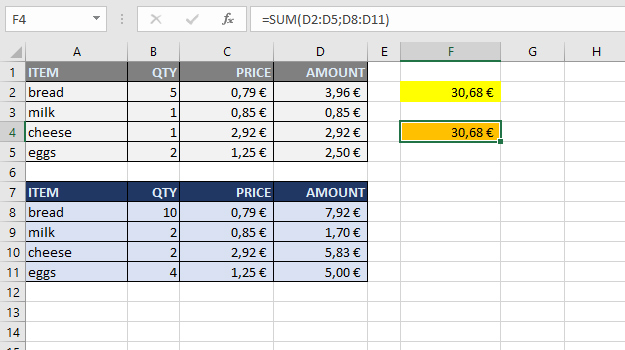# Summarizing in gross

The old word angro, sometimes used in the Serbian language, comes from the French term “en gros” which means “in gross”, “wholesale” … It served me as the inspiration for the title, and in the text I want to show you advanced possibilities when using the SUM function. Let’s see in what, not very common, ways we can give it arguments and summarize the data.

Let’s start with a simple sales table. The easiest way to summarize all the amounts is to write a formula:

=SUM(D2:D5)

However, if we work with a large spreadsheet where it is difficult to select values within amounts column, and knowing that in given column there are no other values than amounts we want to summarize, we could write such formula:

=SUM(D:D)

Assuming that we didn’t enter a formula within a cell in column D it will work perfectly, because we “told” the SUM function to summarize all the values within column D.Let’s copy the previous table with sales data below and make another one, only with double the quantities compared to the first one. As you can see, since we added all the values ​​in column D to the previous formula, it was automatically updated, but we could also use the following formula:

=SUM(D2:D5,D8:D11)

The result is the same. In this formula, the SUM function has two arguments, given as two ranges. We can give it up to 255 arguments and, provided we have entered the correct ones, it will always calculate their sum.The SUM function can be used for summarizing multiple ranges even when contained in different worksheets or documents. Imagine wanting to sum up ranges D2:D5 found in Sheet1, Sheet2 and Sheet3 worksheets. You should write a formula:

=SUM(Sheet1!D2:D5,Sheet2!D2:D5,Sheet3!D2:D5)

Three worksheets is not a problem, but imagine if there is more of them? If you are summarizing ranges within consecutive worksheets you could enter a formula in such manner:

=SUM(Sheet1:Sheet3!D2:D5)

It will summarize all D2:D5 ranges from Sheet1 to Sheet3.